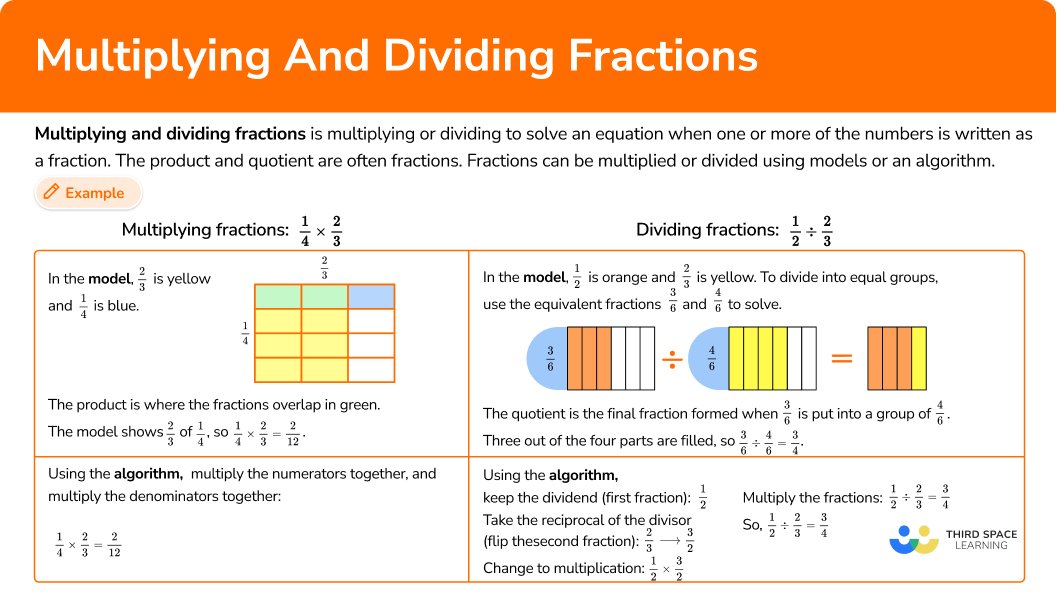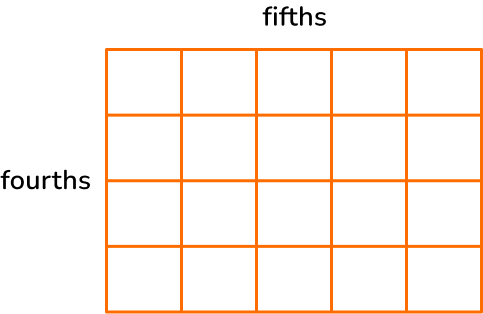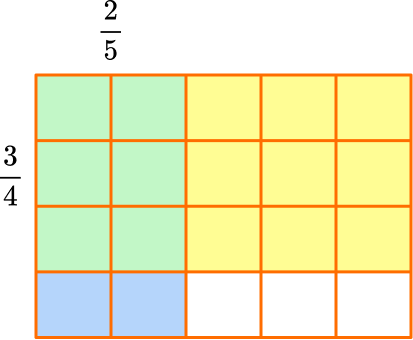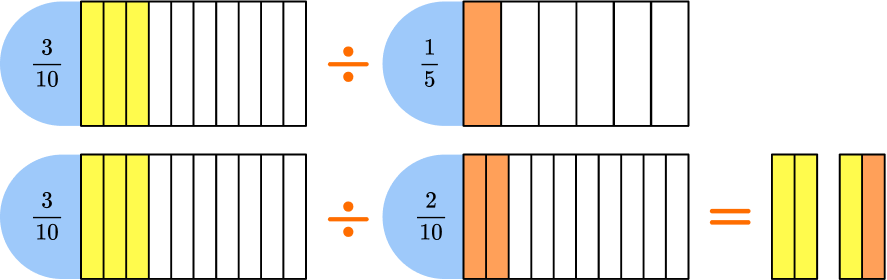# Multiplying and dividing fractions

Here you will learn about multiplying and dividing fractions, including calculations involving whole numbers, proper and improper fractions, and mixed numbers.

Students will first learn about multiplying and dividing fractions as a part of number and operations in fractions in elementary school. They continue to build on this knowledge as a part of the number system in middle school.

## What is multiplying and dividing fractions?

Multiplying and dividing fractions is multiplying or dividing to solve an equation when one or more of the numbers is written as a fraction. The product and quotient are often fractions. Fractions can be multiplied or divided using models or an algorithm.

For example,

\cfrac{1}{4} \times \cfrac{2}{3}

Using models:

In the model, \cfrac{2}{3} is yellow and \cfrac{1}{4} is blue.

The product is where the fractions overlap in green.

The model shows \cfrac{2}{3} of \cfrac{1}{4}, so \cfrac{1}{4} \times \cfrac{2}{3}=\cfrac{2}{12}.

Using the algorithm:

To multiply fractions, you multiply the numerators together, and multiply the denominators together: \cfrac{1}{4} \times \cfrac{2}{3}=\cfrac{2}{12}.

You can also divide fractions with a model or an algorithm.

For example,

\cfrac{1}{2}\div \cfrac{2}{3}

Using models:

Think of this equation as how many \cfrac{2}{3} fit into \cfrac{1}{2}.

In the model, \cfrac{1}{2} is orange and \cfrac{2}{3} is yellow.

To divide into equal groups, use the equivalent fractions \cfrac{3}{6} and \cfrac{4}{6}.

The quotient is the final fraction formed when \frac{3}{6} is put into a group of \cfrac{4}{6}.

Three out of the four parts are filled, so \cfrac{3}{6}\div \cfrac{4}{6} = \cfrac{3}{4}.

Using the algorithm:

KEEP the first fraction, FLIP the second fraction, CHANGE to multiplication.

\cfrac{1}{2} \div \cfrac{2}{3}

Keep the dividend (first fraction): \cfrac{1}{2}

Take the reciprocal of the divisor (flip the second fraction): \cfrac{2}{3} → \cfrac{3}{2}

Change to multiplication: \cfrac{1}{2} \times \cfrac{3}{2}

Multiply the fractions: \cfrac{1}{2} \times \cfrac{3}{2}=\cfrac{3}{4}

\cfrac{1}{2} \div \cfrac{2}{3}=\cfrac{3}{4}

Since \cfrac{1}{2} is smaller than \cfrac{2}{3}, the answer makes sense. A smaller number divided by a larger number, will have a quotient of less than 1.

Note that, unlike when you add and subtract fractions, you do not need a common denominator to multiply and divide fractions when using the algorithm.

Step-by-step guide: Multiplying fractions

Step-by-step guide: Dividing fractions

### What is multiplying and dividing fractions?## Common Core State Standards

How does this relate to 4th grade math and 5th grade math?

• Grade 4 – Number and Operations – Fractions (4.NF.B.4b)
​​Understand a multiple of \cfrac{a}{b} as a multiple of \cfrac{1}{b}, and use this understanding to multiply a fraction by a whole number.

• Grade 5 – Number and Operations – Fractions (5.NF.B.4b)
Apply and extend previous understandings of multiplication to multiply a fraction or whole number by a fraction.

• Grade 6 – Number System (6.NS.A)
Apply and extend previous understandings of multiplication and division to divide fractions by fractions.

## How to multiply with fractions

In order to multiply fractions with models:

1. Draw one fraction horizontally and the other vertically.
2. Connect the fractions all the way across with 2 different colors.
3. Count the overlap.
4. If possible, simplify or convert to a mixed number.

In order to multiply fractions, whole numbers and mixed numbers with the algorithm:

1. Convert whole numbers and mixed numbers to improper fractions.
2. Multiply the numerators together.
3. Multiply the denominators together.
4. If possible, simplify or convert to a mixed number.

In order to solve multiplying fraction word problems:

1. Create an equation to model the problem.
2. Convert whole numbers and mixed numbers to improper fractions.
3. Multiply the numerators together.
4. Multiply the denominators together.
5. If possible, simplify or convert to a mixed number.

## Multiplying and dividing fractions examples

### Example 1: multiplying a fraction by a fraction with a model

Solve \cfrac{3}{5} \times \cfrac{1}{8} with a model.

1. Draw one fraction horizontally and the other vertically.

Draw a rectangle. Divide one side into fifths.

Divide the other side into eighths.

2Connect the fractions all the way across with 2 different colors.

Shade in \cfrac{3}{5} with yellow and \cfrac{1}{8} with blue.

3Count the overlap.

The product is where the fractions overlap in green.

The model shows \cfrac{1}{8} of \cfrac{3}{5}, so \cfrac{3}{5} \times \cfrac{1}{8}=\cfrac{3}{40}, because there are 3 green squares and the whole has 40 squares in total.

4If possible, simplify or convert to a mixed number.

3 and 40 only have a common factor of 1, so the fraction cannot be simplified.

So, \; \cfrac{3}{5} \times \cfrac{1}{8}=\cfrac{3}{40}

### Example 2: multiplying a fraction by a fraction with the algorithm

Solve \cfrac{1}{3} \times \cfrac{5}{6}.

There are no whole numbers or mixed numbers that need to be converted.

\cfrac{1}{3} \times \cfrac{5}{6}=\cfrac{5}{}

\cfrac{1}{3} \times \cfrac{5}{6}=\cfrac{5}{18}

5 and 18 only have a factor of 1, so the fraction cannot be simplified.

So, \cfrac{1}{3} \times \cfrac{5}{6}=\cfrac{5}{18}.

### Example 3: multiplying a whole number by a fraction with the algorithm

Solve \cfrac{6}{10} \times 3.

Write 3 as an improper fraction, 3=\cfrac{3}{1}.

\cfrac{6}{10} \times \cfrac{3}{1}=\cfrac{18}{}

\cfrac{6}{10} \times \cfrac{3}{1}=\cfrac{18}{10}

The numerator is greater than the denominator, so the improper fraction can be converted to a mixed number.

\cfrac{18}{10}=1 \cfrac{8}{10}

The product can be simplified. Both 8 and 10 have a common factor of 2.

\cfrac{8 \; \div \; 2}{10 \; \div \; 2}=\cfrac{4}{5}

So, \cfrac{6}{10} \times \cfrac{3}{1}=1 \cfrac{4}{5}.

### Example 4: multiplying a mixed number by a fraction with the algorithm

Solve \cfrac{5}{12} \times 2 \cfrac{3}{4}.

Convert the mixed number to an improper fraction.

2 \cfrac{3}{4}=\cfrac{11}{4}

\cfrac{5}{12} \times \cfrac{11}{4}=\cfrac{55}{}

\cfrac{5}{12} \times \cfrac{11}{4}=\cfrac{55}{48}

The numerator is greater than the denominator, so the improper fraction can be converted to a mixed number.

\cfrac{55}{48}=1 \cfrac{7}{48}

The product cannot be simplified. 7 and 48 only have a common factor of 1.

So, \cfrac{5}{12} \times \cfrac{11}{4}=\cfrac{55}{48} or 1 \cfrac{7}{48}.

### Example 5: word problem multiplying with fractions

Mr. Sanchez has a rectangular patio in his back yard that measures 2 \cfrac{2}{5}m by 4 \cfrac{1}{2}m. What is the area of Mr. Sanchez’s patio?

Area of a rectangle is l \times w, so use 2 \cfrac{2}{5} \times 4 \cfrac{1}{2} to solve.

Convert the mixed numbers to improper fractions.

\cfrac{12}{5} \times \cfrac{9}{2}=\cfrac{108}{}

\cfrac{12}{5} \times \cfrac{9}{2}=\cfrac{108}{10}

The numerator is greater than the denominator, so the improper fraction can be converted to a mixed number.

\cfrac{108}{10}=10 \cfrac{8}{10}

The product can be simplified. 8 and 10 have a common factor of 2.

\cfrac{8 \; \div \; 2}{10 \; \div \; 2}=\cfrac{4}{5}

So, \cfrac{12}{5} \times \cfrac{9}{2}=\cfrac{108}{10} or 10 \cfrac{4}{5}.

## How to divide with fractions

In order to divide fractions, whole numbers, and mixed numbers with the algorithm:

1. Change the whole numbers and mixed numbers to improper fractions.
2. Take the reciprocal (flip) of the divisor (second fraction).
3. Change the division sign to a multiplication sign.
4. Multiply the fractions together.
5. If possible, simplify or convert to a mixed number.

In order to solve dividing fraction word problems:

1. Create an equation to model the problem.
2. Change any mixed numbers to an improper fraction.
3. Take the reciprocal (flip) of the divisor (second fractions).
4. Change the division sign to a multiplication sign.
5. Multiply the fractions together.
6. If possible, simplify or convert to a mixed number.

### Example 6: dividing a fraction by a fraction

Divide the numbers \cfrac{1}{3} \div \cfrac{1}{6}.

\cfrac{1}{6} → \cfrac{6}{1}

\cfrac{1}{3} \times \cfrac{6}{1}

\cfrac{1}{3} \times \cfrac{6}{1}=\cfrac{6}{3}

\cfrac{6}{3}=2.

This can also be solved with a model.

You can think of this equation as how many \cfrac{1}{6} fit into \cfrac{1}{3}.

In the model, \cfrac{1}{3} is yellow and \cfrac{1}{6} is orange.

To divide into equal groups, the fractional pieces need to be the same size.

Use \cfrac{2}{6} and \cfrac{1}{6} to solve.

The quotient is the final fraction formed when \cfrac{2}{6} is put into groups of \cfrac{1}{6}.

Two groups of \cfrac{1}{6} are in \cfrac{2}{6}, so \cfrac{2}{6} \div \cfrac{1}{6}=2.

### Example 7: dividing a fraction by a whole number

Divide the numbers \cfrac{1}{5} \div 4.

\cfrac{4}{1} → \cfrac{1}{4}

\cfrac{1}{5} \times \cfrac{1}{4}

\cfrac{1}{5} \times \cfrac{1}{4}=\cfrac{1}{20}

\cfrac{1}{20} is in its simplest form and cannot be changed into a mixed number.

This can also be solved with a model. \cfrac{1}{5} divided into equal groups of 4 creates twentieths.

### Example 8: dividing a mixed number by a mixed number

Divide the numbers 2 \cfrac{3}{4} \div 1 \cfrac{1}{12}.

\cfrac{13}{12} \cfrac{12}{13}

\cfrac{11}{4} \times \cfrac{12}{13}

\cfrac{11}{4} \times \cfrac{12}{13}=\cfrac{132}{52}

The numerator is greater than the denominator, so the improper fraction can be converted to a mixed number.

\cfrac{132}{52}=2 \cfrac{28}{52}

The fraction can be simplified. Both 28 and 52 have a factor of 4.

\cfrac{28 \; \div \; 4}{52 \; \div \; 4}=\cfrac{7}{13}

So, \cfrac{11}{4} \times \cfrac{12}{13}=\cfrac{132}{52} or 2 \cfrac{7}{13}.

### Example 9: word problem dividing with fractions

Each cupcake needs \cfrac{1}{4} cup of icing. How many cupcakes can be decorated with 12 cups of icing?

12 \div \cfrac{1}{4}= \; ?

The whole number 12 should be changed to an improper fraction.

12=\cfrac{12}{1}

\cfrac{1}{4} → \cfrac{4}{1}

\cfrac{12}{1} \times \cfrac{4}{1}

\cfrac{12}{1} \times \cfrac{4}{1}=\cfrac{48}{1}

\cfrac{48}{1}=48 which is a whole number.

12 cups of icing will decorate 48 cupcakes.

### Teaching tips for multiplying and dividing fractions

• For elementary students, multiplying and dividing fractions can be a challenging topic. This type of multiplication and division does not always lend itself well to the idea of repeated addition or sharing into equal groups, which are ideas students rely on for whole number multiplication and division. As much as possible, use hands-on visual models and/or digital models so that students can understand what multiplication and division of fractions is. Taking time to do this in the beginning will benefit students when they begin to encounter word problems and real world situations with fractions.

• Highlight patterns within and between multiplication and division of fractions as students are learning. Encourage students to be pattern-seekers themselves. This will help them make sense of the steps involved in multiplying and dividing fractions and understand why the algorithms work.

• Use a variety of activities when teaching multiplying and dividing fractions, instead of relying on just practice worksheets.

### Our favorite mistakes

• Finding the common denominator
When multiplying or dividing fractions with the algorithm, you do not need to find a common denominator. Finding one won’t necessarily lead to the wrong answer, but it creates extra work and leaves more room for mistakes.
For example,
No common denominator created: Common denominator created:

• Using the reciprocal when multiplying
For fraction multiplication, do not flip the second fraction (use the reciprocal) like when dividing. It will lead to the wrong answer.
For example,

• Cross multiplying
If students have been introduced to cross multiplying, they may get confused and use it when multiplying or dividing two fractions.
For example,

• Taking the reciprocal of the dividend (first fraction)
When using the division algorithm, it is the divisor (second fraction) that becomes the reciprocal, not the dividend (first fraction).
For example,

• Not changing the operation to multiplication
When dividing, the operation needs to be changed to multiplication when solving with the reciprocal of the divisor.
For example,

### Practice multiplying and dividing fractions questions

1. Solve \cfrac{3}{4} \times \cfrac{2}{5}.

\cfrac{15}{8}\cfrac{6}{20}\cfrac{23}{20}\cfrac{5}{9}To solve using a model, draw a rectangle. Divide one side into fourths.

Divide the other side into fifths.Shade in \cfrac{3}{4} with yellow and \cfrac{2}{5} with blue.The product is where the fractions overlap in green.

The model shows \cfrac{2}{5} of \cfrac{3}{4}, so \cfrac{3}{4} \times \cfrac{2}{5}=\cfrac{6}{20}, because there are 6 green squares and the whole has 20 squares in total.

The product can be simplified.

Both 6 and 20 have a factor of 2, so they can be divided by 2\text{:} \; \cfrac{6 \; \div \; 2}{20 \; \div \; 2}=\cfrac{3}{10}.

So, \cfrac{3}{4} \times \cfrac{2}{5}=\cfrac{6}{20} or \cfrac{3}{10}.

2. Solve \cfrac{3}{8} \times \cfrac{4}{10}.

\cfrac{30}{32}\cfrac{7}{18}\cfrac{62}{80}\cfrac{12}{80}Multiply the numerators together: \cfrac{3}{8} \times \cfrac{4}{10}=\cfrac{12}{}.

Then, multiply the denominators together: \cfrac{3}{8} \times \cfrac{4}{10}=\cfrac{12}{80}.

The product can be simplified. 12 and 80 have a common factor of 4, so they can each be divided by 4\text{:} \; \cfrac{12 \; \div \; 4}{80 \; \div \; 4}=\cfrac{3}{20}.

So, \cfrac{3}{8} \times \cfrac{4}{10}=\cfrac{12}{80} or \cfrac{3}{20}.

3. Solve 3 \times \cfrac{4}{6}.

\cfrac{18}{4}\cfrac{7}{6}\cfrac{12}{6}\cfrac{4}{18}Write 3 as an improper fraction, 3=\cfrac{3}{1}.

Then, multiply the numerators together: \cfrac{3}{1} \times \cfrac{4}{6}=\cfrac{12}{}.

Then, multiply the denominators together: \cfrac{3}{1} \times \cfrac{4}{6}=\cfrac{12}{6}.

The numerator is greater than the denominator, so the improper fraction can be converted to a mixed number.

\cfrac{12}{6}=2

So, \cfrac{3}{1} \times \cfrac{4}{6}=\cfrac{12}{6} or 2.

4. Solve 2 \cfrac{5}{12} \times 1 \cfrac{1}{2}. Write the product as a mixed number in lowest terms.

3 \cfrac{5}{8}3 \cfrac{2}{12}2 \cfrac{17}{18}5 \cfrac{13}{15}Convert the mixed numbers to improper fractions.Then, multiply the numerators together: \cfrac{29}{12} \times \cfrac{3}{2}=\cfrac{87}{}.

Then, multiply the denominators together: \cfrac{29}{12} \times \cfrac{3}{2}=\cfrac{87}{24}.

The numerator is greater than the denominator, so the improper fraction can be converted to a mixed number.

\cfrac{87}{24}=3 \cfrac{15}{24}

The product can be simplified.

Both 15 and 24 have a factor of 3, so they can be divided by 3\text{:} \; \cfrac{15 \; + \; 3}{24 \; \div \; 3}=\cfrac{5}{8}.
So, \cfrac{29}{12} \times \cfrac{3}{2}=\cfrac{87}{24} or 3 \cfrac{5}{8}.

5. Solve \cfrac{3}{10} \div \cfrac{1}{5}. Write the product in lowest terms.

\cfrac{5}{10}\cfrac{1}{2}\cfrac{2}{3}1 \cfrac{1}{2}Keep the dividend (first fraction): \cfrac{3}{10}

Take the reciprocal of the divisor (flip the second fraction): \cfrac{1}{5} → \cfrac{5}{1}

Change to multiplication: \cfrac{3}{10} \times \cfrac{5}{1}

Multiply the fractions: \cfrac{3}{10} \times \cfrac{5}{1}=\cfrac{15}{10}

Simplify and convert to a mixed number: \cfrac{15}{10}=1 \cfrac{5}{10}=1 \cfrac{1}{2}

This can also be solved with a model.

You can think of this equation as how many \cfrac{1}{5} fit into \cfrac{3}{10}.

In the model, \cfrac{3}{10} is yellow and \cfrac{1}{5} is orange.

To divide into equal groups, the fractional pieces need to be the same size.

Use \cfrac{3}{10} and \cfrac{2}{10} to solve.

The quotient is the final fraction formed when \cfrac{3}{10} is put into groups of \cfrac{2}{10}.The three yellow tenths, fit into a group of 2 tenths, with one out of two in the second group, so \cfrac{3}{10} \div \cfrac{2}{10}=1 \cfrac{1}{2}.

6. Solve \cfrac{7}{8} \div 3.

\cfrac{24}{7}\cfrac{8}{21}\cfrac{4}{17}\cfrac{7}{24}Keep the dividend (first fraction): \cfrac{7}{8}

Take the reciprocal of the divisor (flip the second fraction): \cfrac{3}{1} → \cfrac{1}{3}

Change to multiplication: \cfrac{7}{8} \times \cfrac{1}{3}

Multiply the fractions: \cfrac{7}{8} \times \cfrac{1}{3}=\cfrac{7}{24}

Simplify and convert to a mixed number: \cfrac{7}{24} is in its lowest terms.

7. Solve 1\cfrac{5}{6}\div 2\cfrac{2}{3}. Write the product in lowest terms.

\cfrac{11}{16}4 \cfrac{1}{2}4 \cfrac{8}{9}\cfrac{9}{44}Change the mixed numbers to improper fractions:Keep the dividend (first fraction): \cfrac{11}{6}

Take the reciprocal of the divisor (flip the second fraction): \cfrac{8}{3} → \cfrac{3}{8}

Change to multiplication: \cfrac{11}{6} \times \cfrac{3}{8}

Multiply the fractions: \cfrac{11}{6} \times \cfrac{3}{8}=\cfrac{33}{48}

Change back into a mixed number:

In \cfrac{33}{48}, the numerator is less than the denominator, so it will not be a mixed number.

Simplify: \cfrac{33 \; \div \; 3}{48 \; \div \; 3}=\cfrac{11}{16}, so the answer in lowest terms is \cfrac{11}{16}.

8. Jenny is making bracelets. She uses 1 \cfrac{3}{4} \, \text{ft} of string for each bracelet. How many complete bracelets can she make with 20 \, \text{ft} of string?

3 bracelets10 bracelets11 bracelets20 braceletsCreate the equation 20 \div 1 \cfrac{3}{4} = \; ?

Change the mixed numbers to improper fractions:

1 \cfrac{3}{4}=\cfrac{7}{4}Keep the dividend (first fraction): 20 = \cfrac{20}{1}

Take the reciprocal of the divisor (flip the second fraction): \cfrac{7}{4} → \cfrac{4}{7}

Change to multiplication: \cfrac{20}{1} \times \cfrac{4}{7}

Multiply the fractions: \cfrac{20}{1} \times \cfrac{4}{7}=\cfrac{80}{7}

Change back into a mixed number: \cfrac{80}{7}=11 \cfrac{3}{7}

Simplify: \cfrac{3}{7} is in lowest terms, so the answer is 11 \cfrac{3}{7}.

Jenny can make 11 complete bracelets.

## Multiplying and dividing fractions FAQs

How is multiplying and dividing fractions different?

For multiplying fractions, you multiply the numerators together and the denominators together, but you do not take the reciprocal of either fraction. For dividing fractions, you multiply by using the reciprocal of the divisor (the second fraction).

What vocabulary is used for multiplication and division of fractions?

Students should know and use the following vocabulary…
◌ Numerator: the top number
◌ Denominator: the bottom number
◌ Product: the answer when multiplying
◌ Quotient: the answer when dividing

Should the final answer be in lowest terms?

Although you can encourage students to do it, lowest terms is not a requirement for their understanding of this skill. Students can successfully multiply and divide fractions without using the greatest common factor (GCF) to simplify the products to their lowest terms. However, each state has different expectations of when students should use lowest terms. Refer to state standards for clarification.

## Still stuck?

At Third Space Learning, we specialize in helping teachers and school leaders to provide personalized math support for more of their students through high-quality, online one-on-one math tutoring delivered by subject experts.

Each week, our tutors support thousands of students who are at risk of not meeting their grade-level expectations, and help accelerate their progress and boost their confidence.